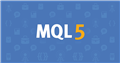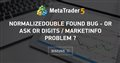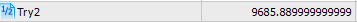# ERROR in MQL NormalizeDouble()

```void OnStart()
{

string x = "9685.894999999";

double y = StringToDouble(x);

double norm_0 = NormalizeDouble(y,0);
double norm_1 = NormalizeDouble(y,1);
double norm_2 = NormalizeDouble(y,2);
double norm_3 = NormalizeDouble(y,3);

Print(norm_0 + " " + norm_1 + " " + norm_2 + " " + norm_3  + " | Original string: " + x + " | StringToDouble " + y );

Print(" beep " + DoubleToString(norm_2,2) );

double yy = 9685.894999999;

double norm_01 = NormalizeDouble(yy,0);
double norm_11 = NormalizeDouble(yy,1);
double norm_21 = NormalizeDouble(yy,2);
double norm_31 = NormalizeDouble(yy,3);

Print(norm_01 + " " + norm_11 + " " + norm_21 + " " + norm_31  + " | Original : " + yy);

Print(" beep " + DoubleToString(norm_21,2) );
}```

# NormalizeDouble

Rounding floating point number to a specified accuracy.

 double  NormalizeDouble(   double  value,      // normalized number   int     digits      // number of digits after decimal point   );
`double norm_21 = NormalizeDouble(yy,2);`

When rounding the float point number with a specified accuracy of 2 digits results in abnormal behavior

```void OnStart()
{

int digit = 2;

double y1 = 9685.891999999;
double n1 = NormalizeDouble(y1,digit);

GlobalVariableSet("y1", n1);

double y2 = 9685.892999999;
double n2 = NormalizeDouble(y2,digit);

GlobalVariableSet("y2", n2);

double y3 = 9685.893999999;
double n3 = NormalizeDouble(y3,digit);

GlobalVariableSet("y3", n3);

double y4 = 9685.894999999;
double n4 = NormalizeDouble(y4,digit);

GlobalVariableSet("y4", n4);

double y5 = 9685.895999999;
double n5 = NormalizeDouble(y5,digit);

GlobalVariableSet("y5", n5);

double y6 = 9685.896999999;
double n6 = NormalizeDouble(y6,digit);

GlobalVariableSet("y6", n6);

double y7 = 9685.897999999;
double n7 = NormalizeDouble(y7,digit);

GlobalVariableSet("y7", n7);

double y8 = 9685.898999999;
double n8 = NormalizeDouble(y8,digit);

GlobalVariableSet("y8", n8);

double y9 = 9685.899999999;
double n9 = NormalizeDouble(y9,digit);

GlobalVariableSet("y9", n9);

Print(" " + n1 +
" " + n2 +
" " + n3 +
" " + n4 +
" " + n5 +
" " + n6 +
" " + n7 +
" " + n8 +
" " + n9
);
}```

Please note that when output to Journal using the Print() function, a normalized number may contain a greater number of decimal places than you expect.

Yes, this is true!  Therefore I make the NormalizedDouble a GlobalVariable and the process of normalization still fails.

If I pass in a value of 1.2345 and only want a precision of Digit(), then how is this possible?Documentation on MQL5: Conversion Functions / NormalizeDouble
• www.mql5.com
Calculated values of StopLoss, TakeProfit, and values of open prices for pending orders must be normalized with the accuracy, the value of which can be obtained by Digits(). Please note...

4 Years ago, this was discussed. However, community members were finding this behavior when Print() the Normalizeddouble.

They were able to solve the issue by converting the double to a string with the same digit precision such as

`DoubleToString(NormalizeDouble(dYourVariable,2), 2);`

Unfortunately, this is not a solution!NormalizeDouble found bug - or Ask or Digits / MarketInfo problem ?
• 2016.05.03
• www.mql5.com
Hello, first of all i have trouble to post on mq4 forum so i write here ... should be same anyways i found a bug...

Print using DoubleToString()

Edit: I see you added a post at the same time as me.

DoubleToString()  IS the solution

Keith Watford:

Print using DoubleToString()

Edit: I see you added a post at the same time as me.

DoubleToString()  IS the solution

Unfortunately, DoubleToString is not a solution when I am not trying to use the Print() function. I only used the Print() function to quickly test the NormalizeDouble function for debugging.

In the 2nd post, I included another script that takes the NormalizedDouble and creates a Global Variable with that value. Unfortunately, the values are identical.

Are there any other ways to "Cut Off" values after the decimal with accurate precision? (Without converting the double to a string)

I don't need to round the value. I only need to make the double accurate with the indicated Digit().Documentation on MQL5: Conversion Functions / NormalizeDouble
• www.mql5.com
Calculated values of StopLoss, TakeProfit, and values of open prices for pending orders must be normalized with the accuracy, the value of which can be obtained by Digits(). Please note...

```  string ss = "9685.89";
double try2 = StringToDouble(ss);
GlobalVariableSet("Try2" , try2);
Print(" TRY 2: " + ss + " " + try2);```

Here is an example of how the StringToDouble adds extra values when converting a string with two values after decimal.torytory:

Here is an example of how the StringToDouble adds extra values when converting a string with two values after decimal.

I don't know what you are trying to do by using a Global Variable or what you are trying to achieve.

torytory:

Unfortunately, DoubleToString is not a solution when I am not trying to use the Print() function. I only used the Print() function to quickly test the NormalizeDouble function for debugging.

You don't have to do anything, once you have normalised a value, that is it, it will be normalised to a value that the computer can store, which means it may not be the exact number of digits that you are expecting after the decimal point.

Keith Watford:

I don't know what you are trying to do by using a Global Variable or what you are trying to achieve.

You don't have to do anything, once you have normalised a value, that is it, it will be normalised to a value that the computer can store, which means it may not be the exact number of digits that you are expecting after the decimal point.

To prove my point that NormalizeDouble is broken. In the documents it states

# NormalizeDouble

Rounding floating point number to a specified accuracy.

The specified accuracy doe not work. What I am trying to do is return a floating point number with a specific accuracy with the exact number and with the exact precision. Even if I were to make the correct precisions by parsing the strings, once I have the correct value within a string, I can't convert the string back to the double without the mql5 program doing something inconstant. I am looking for a solution. Are there any?

torytory:

I am looking for a solution. Are there any?

To find a solution, you first need a problem.

Keith Watford:

I've pointed out the problem. It's a shame that the moderators don't realize that issues exist and not willing to admit them. The documentation clearly states the use of NormalizeDouble function and when I point out that the function does not accuracy produce the correct result I am told that there is no problem.

Basically what you saying is that NormalizeDouble returns whatever it wants and just live with the inaccuracy.

torytory:

I've pointed out the problem. It's a shame that the moderators don't realize that issues exist and not willing to admit them. The documentation clearly states the use of NormalizeDouble function and when I point out that the function does not accuracy produce the correct result I am told that there is no problem.

Basically what you saying is that NormalizeDouble returns whatever it wants and just live with the inaccuracy.

Keith Watford: